# Referring to the Truss Shown in the Figure. Find :(A) Reaction at D and C(B)Zero Force Members.(C)Forces in Member Fe and Dc by Method of Section.(D)Forces in Other Members by Method of Joints. - Engineering Mechanics

Referring to the truss shown in the figure. Find :
(a) Reaction at D and C
(b)Zero force members.
(c)Forces in member FE and DC by method of section.
(d)Forces in other members by method of joints.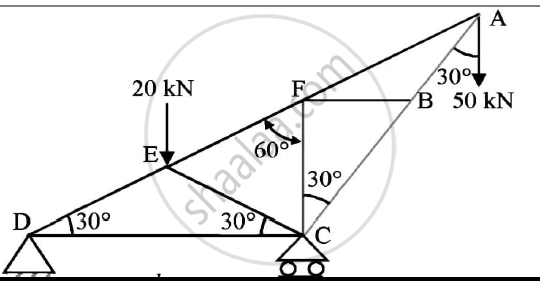#### Solution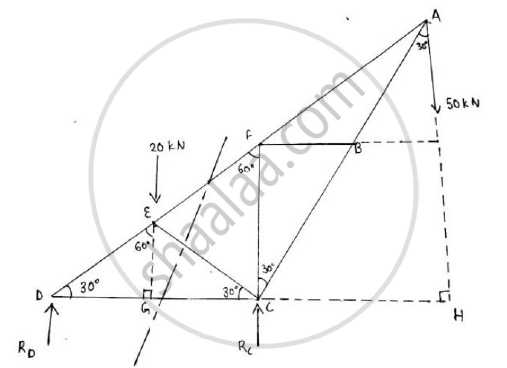By Geometry:
AC = CD = l
Similarly, in Δ EDC,
ED = EC
Δ DEG and Δ CEG are congruent
DG = GC =1/2

In Δ DEG, ∠EDG=30o, ∠DGE=90o
tan30= (EG)/(DG)

EG = DG.tan30 =I/2x1/sqrt3=I/(2sqrt3)
In Δ ACH,
CH =(AC)/2=I/2

DH = DC + CH = l +I/2=(3I)/2
No horizontal force is acting on the truss, so no horizontal reaction will be present at point A The truss is in equilibrium Applying the conditions of equilibrium
Σ MD = 0
-20 x DG -50 x DH + RC x DC = 0
-20 x1/(2)50X(3I)/2+RCX1=0

-10 - 75 + RC = 0
RC = 85 kN
Σ Fy=0
-20 – 50 + RD + RC=0
RD = -15kN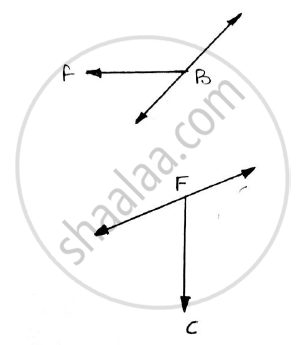As per the rule,member BF will have zero force and is a zero force number. Similarly,Member CF will have zero force
Method of sections :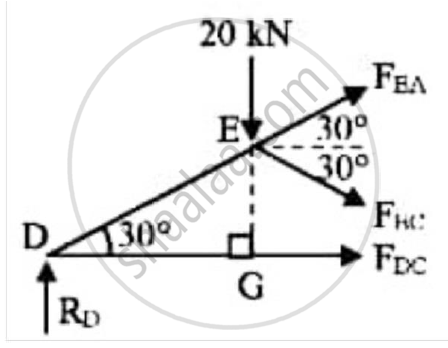Applying the conditions of equilibrium to the section shown
Σ MD = 0
-20 x DG – FECcos 30 x EG - FECsin30 x DG = 0
-20 x I/2 x - FECcos30 x EG - FECsin30 x DG = 0
-20 xI/2x -FEC x sqrt3/2x(I/2)-FEC x1/2XI/2=0
-10 x l - FEC xI/4-FEC xI/4=0
-(2I)/4F_(EC)=10L
FEC = -20kN

RD – 20 - FECsin30 + FEAsin30 = 0
-15 - 20 + 20 x 0.5 + FEA x 0.5 = 0
FECcos30 + FEAcos30 + FDC = 0
-20 x 0.866 + 50 x 0.866 + FDC = 0
FDC = - 25.9808kN

Method of joints: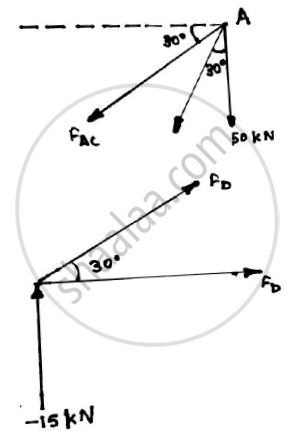Joint A
-50 - FAEsin30 - FACcos30 = 0
-50 - 50 x 0.5 = FAC x 0.866
FAC = -86.6025kN
Joint D
FDC + FDEcos30 = 0
-25.9808 + 0.866FDE = 0
FDE = 30kN

 Member Magnitude (in kN) Nature AE (AF and EF) 50 Tension AC (AB and BC) 86.6025 Compression EC 20 Compression DE 30 Tension DC 25.9808 Compression FB 0 FC 0
Concept: Analysis of Plane Trusses by Using Method of Joints
Is there an error in this question or solution?